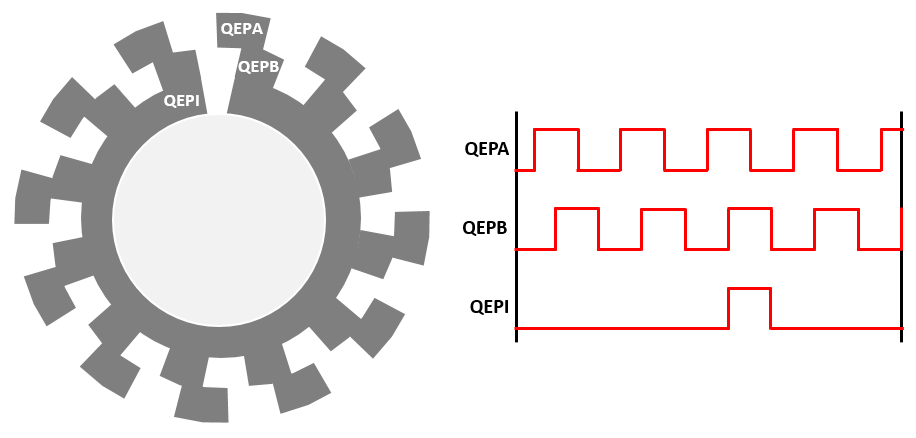• Library:
• Motor Control Blockset / Sensor Decoders

•## Description

The Quadrature Decoder block computes the position of the quadrature encoder. The block uses the current encoder counter value and encoder counter value at the previous index pulse to calculate the angular position of the quadrature encoder (and the rotor) in either degrees, radians, or per-unit.

This figure shows a quadrature encoder disk with two channels (QEPA and QEPB) and an index pulse (QEPI):In this example, the timer driven by the QEP increments by four for each slit:### Equations

The block computes the angular position (in counts) of the quadrature encoder as:

When the encoder rotates in the clockwise direction:

• If $Idx\le Cnt$,

• If $Idx>Cnt$ and the shaft rotation direction does not change,

• If $Idx>Cnt$ and the shaft rotation direction reverses,

When the encoder rotates in the anticlockwise direction:

• If $Idx\ge Cnt$,

• If $Idx and the shaft rotation direction does not change,

• If $Idx and the shaft rotation direction reverses,

When you clear the External index count parameter, the Idx pulse resets Cnt to zero, therefore:

where:

• is the angular position of the quadrature encoder in counts.

• is the number of counts in one rotation cycle of the quadrature encoder.

The block computes the output θm as:

(in degrees)

(in per-unit)

## Ports

### Input

expand all

Value that the quadrature encoder counter generates with respect to the slit-position. The port only accepts a scalar unsigned integer based on the Counter size parameter. For example, if you select `8 bits` for Counter size, the input data type must be uint8.

Data Types: `uint8` | `uint16` | `uint32`

Value that the quadrature encoder counter generated with respect to the slit-position at the time of the last index pulse. The port only accepts a scalar unsigned integer based on the Counter size parameter. For example, if you select `8 bits` for Counter size, the input data type must be uint8.

#### Dependencies

To enable this port, select the External index count parameter.

Data Types: `uint8` | `uint16` | `uint32`

### Note

The input data types for both `Cnt` and `Idx` must be identical.

### Output

expand all

Angular position that the block computes based on the `Cnt` and `Idx` inputs.

Data Types: `single` | `double` | `fixed point`

## Parameters

expand all

The number of slits available in each phase of the quadrature encoder.

The number of counts that the quadrature encoder generates for every slit. A count indicates a slit position. For example, select 4 if you want the encoder to generate four counts corresponding to 00, 10, 11, and 01 slit positions or values.

Size of the quadrature encoder counter.

The block enables the `Idx` input port only if you select this parameter. The block expects that the `Cnt` input port value resets at the time of the `Idx` pulse.

Unit of the angular position output.

The data type for the angular position output.

### Note

The Quadrature Decoder block may occasionally display the warning message `'Wrap on overflow detected`.`'`